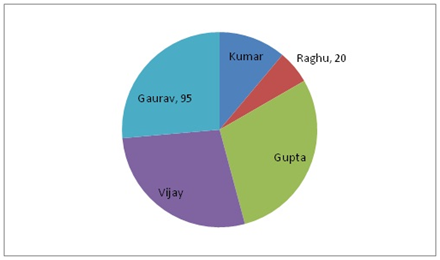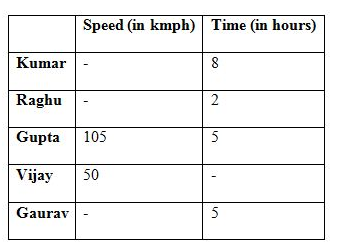# SBI Clerk Mains Quantitative Aptitude (Day-53)

Dear Aspirants, Our IBPS Guide team is providing new series of Quantitative Aptitude Questions for SBI Clerk Mains 2020 so the aspirants can practice it on a daily basis. These questions are framed by our skilled experts after understanding your needs thoroughly. Aspirants can practice these new series questions daily to familiarize with the exact exam pattern and make your preparation effective.

Start Quiz

Direction (1-5): In each of the following questions, two equations are given. You have to solve both the equations to find the relation between x and y.

1) I: 5x2 – 26x + 33 = 0

II: 7y2 – 54y + 99 = 0

A) If x < y

B) If x > y

C) If x ≤ y

D) If x ≥ y

E) If relationship between x and y cannot be determined

2) I: 3x2 – 23x + 40 = 0

II: 15y2 – 34y + 15 = 0

A) If x ≤ y

B) If x ≥ y

C) If relationship between x and y cannot be determined

D) If x < y

E) If x > y

3) I: 8x– 22x + 15 = 0

II: 3y+ 14y + 15 = 0

A) If x > y

B) If x < y

C) If x ≤ y

D) If relationship between x and y cannot be determined

E) If x ≥ y

4)  I: 3x2 + 17x + 24 = 0

II: y2+ 11y + 24 = 0

A) If x < y

B) If x > y

C) If x ≤ y

D) If x ≥ y

E) If relationship between x and y cannot be determined

5) I: 3x2 – 25x + 52 = 0

II: 2y2 – 19y + 35 = 0

A) If relationship between x and y cannot be determined

B) If x > y

C) If x < y

D) If x ≤ y

E) If x ≥ y

Data Interpretation

Direction (6-10): Study the following information carefully and answer the questions given below.

The pie-chart shows the distribution in degree of distance travelled by five boys out of total distance of 1800 km. Some values are missing here.The table shows the average speed and time taken by these five boys while covering the distance.6) If the speed of Kumar is 50% less than the speed of Raghu, then the distance travelled by Kumar is approximately what percent more/less than that of Gupta?

A) 44%

B) 53%

C) 62%

D) 78%

E) 85%

7) Find the time taken by Vijay if it is known that the distance travelled by Kumar is 8/21 of the distance travelled by Gupta?

A) 5 hours

B) 10 hours

C) 15 hours

D) 12 hours

E) None of these

8) Time taken by Vijay is how much more/less than the time taken by Raghu if it is known that the speed of Kumar is same as the speed of Raghu?

A) 2 hours

B) 3 hours

C) 4 hours

D) 5 hours

E) None of these

9) A train, which is at a distance of 3200 km from Gaurav starts approaching towards Gaurav with a speed of x kmph. After 8 hours from the start of train, Gaurav also starts to move towards train at his normal speed. If they meet after 10 hours after Gaurav had started to move, then find the x?

A) 100 kmph

B) 125 kmph

C) 140 kmph

D) 130 kmph

E) None of these

10) If Gupta had travelled half a distance at a speed of y kmph and remaining at a speed of 90 kmph, then find the value of y?

A) 110 kmph

B) 115 kmph

C) 123 kmph

D) 126 kmph

E) None of these

Directions (1-5) :

From I

=> 5x2 – 26x + 33 = 0

=> 5x2 – 15x – 11x + 33 = 0

=> 5x(x – 3) – 11(x – 3) = 0

=> (x – 3) (5x – 11) = 0

=> x = 3, 11/5

From II

=> 7y2 – 54y + 99 = 0

=> 7y2 – 21y – 33y + 99 = 0

=> 7y(y – 3) – 33(y – 3) = 0

=> (y – 3) (7y – 33) = 0

=> y = 3, 33/7

Hence, x ≤ y

From I

=> 3x2 – 23x + 40 = 0

=> 3x2 – 15x – 8x + 40 = 0

=> 3x(x – 5) – 8(x – 5) = 0

=> (3x – 8) (x – 5) = 0

=> x = 5, 8/3

From II

=> 15y2 – 34y + 15 = 0

=> 15y2 – 9y – 25y + 15 = 0

=> 3y (5y – 3) – 5 (5y – 3) = 0

=> (3y – 5) (5y – 3) = 0

=> y = 5/3, 3/5

Hence, x > y

From I

=> 8x– 22x + 15 = 0

=> 8x2 – 12x – 10x + 15 = 0

=> 4x (2x – 3) – 5(2x – 3) = 0

=> (4x – 5) (2x – 3) = 0

=> x = 5/4, 3/2

From II

=> 3y+ 14y + 15 = 0

=> 3y2 + 9y + 5y + 15 = 0

=> 3y(y + 3) + 5(y + 3) = 0

=> (y + 3) (3y + 5) = 0

=> y = -3, -5/3

Hence, x > y

From I

=> 3x2 + 17x + 24 = 0

=> 3x2 + 9x + 8x + 24 = 0

=> 3x(x + 3) + 8(x + 3) = 0

=> (x + 3) (3x + 8) = 0

=> x = -3, -8/3

From II

=> y2+ 11y + 24 = 0

=> y+ 3y + 8y + 24 = 0

=> (y + 3) (y + 8) = 0

=> y = -3, -8

Hence, x ≥ y

From I

=> 3x2 – 25x + 52 = 0

=> 3x2 – 12x – 13x + 52 = 0

=> 3x(x – 4) – 13(x – 4) = 0

=> (3x – 13) (x – 4) = 0

=> x = 13/3, 4

From II

=> 2y2 – 19y + 35 = 0

=> 2y2 – 14y – 5y + 35 = 0

=> 2y(y – 7) -5(y – 7) = 0

=> (2y – 5) (y – 7) = 0

=> y = 5/2, 7

Hence, relationship between x and y cannot be determined.

Directions (6-10) :

Distance covered by Raghu = 1800/360 * 20 = 100 km

Speed of Raghu = 100/2 = 50kmph

Speed of Kumar = 50% of 50 = 25 kmph

Required percentage

= (105 * 5 – 25 * 8)/(105 * 5) * 100 = 61.90% ≈ 62%

Hence, the required answer = 62%

Distance travelled by Kumar = 8/21 * 105 * 5 = 200 km

Distance travelled by Raghu = 1800/360 * 20 = 100 km

Distance travelled by Gupta = 105 * 5 = 525 km

Distance travelled by Gaurav = 1800/360 * 95 = 475 km

Distance travelled by Vijay = 1800 – 200 – 100 – 525 – 475 = 500 km

Time taken by Vijay = 500/50 = 10 hours

Hence, the required answer = 10 hours

Distance covered by Raghu = 1800 * 20/360 = 100km

Time taken by Raghu = 2 hours

Speed of Raghu = 100/2 = 50 kmph

Speed of Kumar = 50 kmph

Distance covered by Vijay

= 1800 – 50 * 8 – 50 * 2 – 105 * 5 – 95 * 5 = 300 km

Time taken by Vijay = 300/50 = 6 hours

Required time difference = 6 – 2 = 4 hours

Hence, the required answer = 4 hours

Speed of train = x kmph

In 8 hours, it would have covered 8x km.

Speed of Gaurav = Distance/Time=> 3200 – 8x = 950 + 10x

=> 18x = 3200 – 950

=> x = 125kmph

Hence, the required answer = 125 kmph

Average speed of Gupta = 105 kmph

Distance travelled by Gupta = 105 * 5 = 525 km525 (90y) = 105 (262.5 * 90 + 262.5 * y)

450y = 23625 + 262.5y

187.5 y = 23625

=> y = 126 kmph

Hence, the required answer = 126 kmph

 Check Here to View SBI Clerk Mains 2020 Quantitative Aptitude Questions Day 52 Day 51 Day 50 Click Here for SBI Clerk 2020 – Detailed Exam Notification